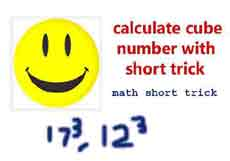# How to calculate the cube root with short trickToday we are display the short cut for calculate  the cube root with short cut. Number of question put for this topic in all competitive exams SSC CGL clerk Bank PO Clerk CAT MAT Army clerk Delhi police and other defence exam , and also common topic in 10th class 12th class .Now we are display the calculate the cube root with short trick in simple way and solve the this type question with in second .

If we are solved the cube root with basic formula so maximum time consume in competitive exams.

We are display the 2 method

Daily Visit GK on myshort.in

1 Method=(22) = 484(20+2)=9680+968 = 10648=(39) = 1521(30+9)= 45630+13689 = 59319=(18)= 324(10+8)=3240 + 2592=5832

2 Method1 carry 18 4 8 1 7 2 8

last digit or unit value= 2,= 4,= 8

Multiple 2 only middle value

4*2 =8

8+4=12  write only 2 and 1 is carry and add in next value

1+2+4=7

Explain

Step 1. Select the last number or unit place of the value

in case unit place or last number of 12  is 2

Step 2.

write the unit place in this way

first time write unit place simple=  2

2nd time squarer the unit place= 4

3rd time cube root of unit place= 8

Step 3

now always multiple by 2 for middle 2 digits shoe in calculation

2*2 = 4

4*2 = 8

Step 4

Add the number show in calculation

Not we are used carry word meaning that

4+8 = 12 so write the only 2 and 1 is carry

Question1 5 carry 6 carry 164 8 32 2 7 5 4 4

Final result = 2744

in 3rd column  solve

6+16+32=  54

6+6+2 = 14  write only 4 and one carry

1+1+3=5Question

 3 18 34 1343 14 98 4 9 1 3

Ans 4913

Step 1  select in 343 = 3 and carry 34

Step 2 Now 34+49+98=

4+9+8=21  and now write 1 and carry 2

2+3+4+9=18 carry and add in next value.

Step3   Now 18+7+14 =

8+7+4=19  carry 1

1+1+1=3  carry add in next value

Step 4

3+1=4

Buy online Rajasthan gk book Railway JE CBT REET PAtwari Book SSC CGL Clerk GD Book Buy online Rajasthan gk book Railway JE CBT REET PAtwari Book SSC CGL Clerk GD Book